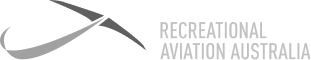# Definition: Lift

A force that opposes weight. If the lift force is more than the weight force, then the aircraft will climb. If it is less than the force of weight, then the aircraft will descend.### Lift

A force that opposes weight. If the lift force is more than the weight force, then the aircraft will climb. If it is less than the force of weight, then the aircraft will descend.

7 Day

FREE

Trial